##### Journal of
###### eISSN: 2473-0831# Analytical & Pharmaceutical ResearchResearch Article Volume 11 Issue 2

# Equilibrium and kinetic behavior of Pb(II) ions sorption onto thermally treated clay-rich soilcollected near Gelemso town of Oromia region in Ethiopia

#### Abraham Fikre, Tesfahun Kebede, OP Yadavfunction clickButton(){ var name=document.getElementById('name').value; var descr=document.getElementById('descr').value; var unCopyslNo=document.getElementById('unCopyslNo').value; document.getElementById("mydiv").style.display = "none"; $.ajax({ type:"post", url:"https://medcraveonline.com/captchaCode/server_action", data: { 'name' :name, 'descr' :descr, 'unCopyslNo': unCopyslNo }, cache:false, success: function (html) { //alert('Data Send');$('#msg').html(html); } }); return false; } Verify Captcha Regret for the inconvenience: we are taking measures to prevent fraudulent form submissions by extractors and page crawlers. Please type the correct Captcha word to see email ID.function refreshPage(){ $("#mydiv").load(location.href + " #mydiv"); }$(document).ready(function () { //Disable cut copy paste $('#msg').bind('cut copy paste', function (e) { e.preventDefault(); }); //Disable mouse right click$("#msg").on("contextmenu",function(e){ return false; }); }); .noselect { -webkit-touch-callout: none; /* iOS Safari */ -webkit-user-select: none; /* Safari */ -khtml-user-select: none; /* Konqueror HTML */ -moz-user-select: none; /* Firefox */ -ms-user-select: none; /* Internet Explorer/Edge */ user-select: none; /* Non-prefixed version, currently supported by Chrome and Opera */ cursor: none; }

Chemistry Department, Haramaya University, Ethiopia

Correspondence: OP Yadav, Chemistry Department, Haramaya University, Ethiopia

Received: April 11, 2022 | Published: June 30, 2022

Citation: Fikre A, Kebede T, Yadav OP. Equilibrium and kinetic behavior of Pb(II) ions sorption onto thermally treated clay-rich soil collected near Gelemso town of Oromia region in Ethiopia. J Anal Pharm Res. 2022;11(3):79-87. DOI: DOI: 10.15406/japlr.2022.11.00405

# Abstract

Equilibrium and kinetic studies of Pb (II) ions sorption onto a thermally treated clay-rich soil, collected from a site (8.822634N, 40.523574E) near Gelemso town in Western Hararghie Zone of Oromia region of Ethiopia, have been carried out by batch experiments. The sorption equilibrium data analyzed in the light of Langmuir-, Freundlich-, and Temkin isotherms showed that the Freundlich isotherm represents the best fit for the experimental data. For revealing the mechanism of Pb (II) ions sorption onto the studied sorbent, the observed data was analyzed in terms of pseudo-first-order, pseudo-second-order, intra-particle diffusion, Boyd, and Elovich Kinetic Models. The pseudo-second-order kinetic model with the high correlation coefficient (R2) predicted sorption capacity closer to the experimental value. With Pb(II) initial concentration 20 mg L-1, and optimized adsorbent dose and pH, as high as 95% Pb(II) ions could be removed within 3 hrs. Therefore, the studied clay-rich soil can be used as a low-cost and efficient alternative sorbent, for the large scale treatment of wastewater contaminated with Pb (II) ions.

Keywords: chemisorption, intra-particle, diffusion, equilibrium, isotherms

# Introduction

The increasing establishment of industries manufacturing various agrochemicals, petrochemicals and diverse anthropogenic activities have led to the pollution of our environment with diverse hazardous chemicals including heavy metals.1,2 Most of the global surface water contaminated with heavy metals has become a serious health problem for living bodies. The hazardous substances including heavy metals present in wastewater endanger the public health as well as aquatic organisms. Additionally, heavy metals-loaded untreated effluents released from some industries degrade the agricultural land causing a diminished crops yield.

The conventional methods including ion-exchange, coagulation, chemical reduction, and precipitation employed for the removal of heavy metals from the contaminated water are expensive as well as inefficient.3,4 Adsorption method, using activated carbon, has been an efficient alternative for the effective removal of heavy metals from wastewater,8,9 but the high cost of the activated carbon limits its application at a small-scale treatment of contaminated water.

However, to reduce the cost of the adsorption process, inexpensive agricultural biowaste material such as groundnut husk,10 rice husk,11,12 pine needles powder,13 guar gum biocomposite,14 coconut waste15 or the carbon derived from these, have earlier been used. Al-Degs et al.16 employed natural sorbents (consisting of primary minerals, i.e., quartz and aluminosilicates and secondary minerals, i.e., calcite and dolomite) for the removal of some heavy metals. Brooks et al.17 used alkali ash material permeable reactive barrier (AAM-PRB), a newly developed material for the removal of lead from contaminated water by batch and column experiments. Hirut et al.18 employed low cost and eco-friendly bio-sorbent eucalyptus camaldulensis to study its capacity for removing toxic heavy metals Pb, Cd and Cr from synthetic and industrial wastewater released from textile and leather industries. Review reports on adsorption phenomenon and removal of heavy metals including Pb have also appeared in the literature.19–24

Low-cost clay-rich soil may be a promising sorbent material for the treatment of wastewater contaminated with heavy metals.25 Mnasri-Ghnimi et al.26 have used single and mixed pillared clays for the removal of some heavy metals from wastewater. In the present study, sorption behavior of Pb (II) ions over a thermally treated clay-rich soil, collected from a site (8.822634N, 40.523574E) near Gelemso town in the Western Hararghie zone of Oromia region of Ethiopia, have been studied.

# Material and methods

Chemicals

Lead(II) nitrate [Pb (NO3)2, MW: 331.2, gmol−1) and hydrochloric acid (HCl, MW: 36.5 gmol−1) were from Blulux Laboratories. Nitric acid (HNO3, 98%, MW:63.10 gmol−1), Sodium hydroxide (NaOH, 98% , MW: 40.0 gmol−1), Sodium Chloride (NaCl, 98% MW:58.44 gmol−1), Phenolphthalein (C₂₀H₁₄O₄, MW: 318.328 gmol−1) were procured from Breckland Scientific Supplies, UK and Oxalic acid (H2C2O4, 99.8%, MW: 90.03 gmol−1), was from BDH Laboratories.

Sorbent material

Soils were collected from three different sites having geographical co-ordinates: (8.909331, 40.772494), (9.082266, 40.864575), (8.822634, 40.523574), situated near Bedesa, Asebe Teferi and Gelemso towns, respectively, in the western Hararghie zone of Oromia region of Ethiopia (Figure 1), and these were coded as: S1, S2 and S3, respectively. Soil sample was collected with the help of an Auger in a zigzag pattern up to 30 cm depth below the surface, from 15 different spots with one meter inter-spot separation and then mixed together in plastic bag to make a composite sample. The soil samples were dried in open air, pulverized and sieved to get particle size < 0.5 mm and then kept in moisture-free atmosphere before further use.Figure 1 Three sampling sites with geographical co-ordinates: (8.909331N, 40.772494E), (9.082266N, 40.864575E), and (8.822634N, 40.523574E) near Bedesa, Asebe Teferi, and Gelemso towns, respectively in western Hararghie Zone of Oromia region of Ethiopia. (West Harargie map under classification of Ethiopia drawn by using GIS software).

Thermal treatment of sorbent

The optimum temperature for thermal treatment of the soil used as sorbent was determined by heating 4 g of the soil for 2 hrs, at 1000C interval, over temperatures range: 200-9000C and recording each time the sorption of Pb(II) ions from solution of known initial concentration.

Measurement of soil pH

To determine pH of soil, 10 g of the air-dried soil, with particle size < 2 mm, was mixed with 25 mL deionized water in a 100 mL beaker, the soil suspension was stirred for 30 minutes and then kept unstirred for 5 minutes. The pH of the clear supernatant liquid was measured using a pH meter (Model MP220, Switzerland) at ambient temperature.

Measurement of cation exchange capacity

The cation exchange capacity (CEC) of soil was determined using ammonium acetate method.27 Typically, 100 mL 1M ammonium acetate aqueous solution (pH = 7.0) was added to 5 g soil taken in a 250 mL beaker, stirred the mixture for five minutes, and then kept as such, for overnight. The suspension was filtered through a deionized water-soaked Whatman No.1 paper. The residue was washed twice with 25 mL portion each of 1M ammonium acetate followed by washing with five successive 20 mL portion each of 10% NaCl sodium chloride solution, and the filtrate was made up to 100 mL. A blank solution was prepared similarly, where no soil was taken. The filtrate was titrated against 0.1N NaOH, and the CEC was obtained using the equation:

(1)

Where, “a” is mL of 0.1N NaOH required for titrating the blank solution; “b” is mL of 0.1N NaOH required for titrating the sample solution; s = mass (g) of air-dried soil taken; N = normality of NaOH (0.1N), and mcf = moisture correction factor (taken as 1.00)

XRD study

An X-ray diffractometer (Brucker Axis), equipped with “Diffrac Plus 2000 Software” was employed for determining the mineral compositions of soil samples used as adsorbents, The X-ray diifraction pattern was recorded over 2θ range from 40 to 600 with 2-theta and time steps 0.0200 and 1 second, respectively.

Specific surface area of sorbent

Specific surface areas of sorbent (Soil) was determined following Sear´s Method.28,29 Typically, to a mixture of 1.5 g soil. and 30 g NaCl, taken in a glass beaker, was added 0.1N HCl with continuous stirring to attain pH 3.0 and the final volume was made up to 150 mL using deionized water. The mixture was titrated against 0.1M NaOH and the specific surface area of the soil was obtained using the following equation.

(2)

where, S = specific surface area (m2g-1) of soil, V = volume (mL) of 0.1M NaOH solution required to titrate the soil suspension from pH 4 to pH 9.

Point of zero charge (pzc) of sorbent

The pH at which a solid surface (in the absence of any specific sorption) with zero net charge is represented by pzc. The pzc values of sorbent was determined as described by Onyango et al.30 Typically, 50 mL each of 0.01M NaCl solution was taken in separate Erlenmeyer flasks and their pH were adjusted to 2, 4, 6, 8, 10 and 12, respectively, by adding required amount of 0.1M HCl or 0.1M NaOH solution. Each solution was mixed with 0.5 g of sorbent and agitated using a shaker for 1 h and then the potential (mV) of each solution was recorded. The zero potential line intersecting the potential versus pH plot gives the pzc value on the pH Axis of the plot.

Sorption study

Sorption of Pb(II) ions onto the sorbent, in aqueous medium, was studied by batch operation method. In a typical run, a known amount of the sorbent was mixed with 100 mL of Pb(II) aqueous solution, of given concentration, in a 250 ml Erlenmeyer flask. The reaction mixture was stirred at 150 rpm for an hour prior to attaining sorption-desorption equilibrium and then centrifuged at 2500 rpm. The supernatant liquid was then analyzed for Pb(II) ion concentration using an atomic absorption spectrophotometer (BUCK) and adopting 3111A standard procedure31 and measuring the absorbance at 217 nm, slit width 0.7 nm, lamp current 5.0 mA, detection limit 0.01 mg/L. Percentage removal of Pb(II) was calculated using the equation:

Percent removal =     (3)

where, C0 and Ce are initial and equilibrium concentrations of Cr(VI) ions (in mgL-1), respectively. Sorption capacity (qt) of the sorbent for Pb(II) ions, at the given reaction time t, was calculated using the equation:

${q}_{t}=\frac{\left({C}_{0}-{C}_{t}\right)V}{m}$     (4)

Where: Co and Ct are metal ions concentrations (mg/L) in the initial stage and at time, respectively; V is volume of the metal ions solution in liter; m is mass (g) of the sorbent taken, and qt is sorption capacity (mg g-1).

Statistical analysis

The sorption data generated was analyzed using one-way ANOVA, LSD test and regression analysis and graphs were generated using the software package STATISTICA (released 8.0) for WINDOWS.

# Results and discussion

Characteristics of sorbent

Clay percent, pH and cation exchange capacity (CEC) of three soil samples collected from different sites are given in Table 1.

 Soil sample site Soil Code Clay Content (%) Soil pH CEC/meq/100gsoil) Bedesa S1 9.08 6.68 57.4 Asebe Teferi S2 21.08 7.22 42.3 Gelemso S3 43.02 8.73 59.2

Table 1 Some characteristic properties of three soils collected from different sites of western Hararghie zone of Oromia region of Ethiopia

*Bedesa (8.909331N, 40.772494E); Asebe Teferi (9.082266N, 40.864575E), and Gelemso (8.822634N, 40.523574E) in Western Hararghie zone of Oromia region of Ethiopia.

Since the soil sample labeled as S3 (collected from Gelemso) has highest clay content as well as cation exchange capacity, among the three collected soil samples, therefore, it was selected as the sorbent in the subsequent part of the present study.

Thermal treatment of sorbent

To optimize the temperature for thermal treatment of sorbent, soil (S3) was heated from 200 to 9000 C in a step of 100 0C. The plot of percent removal of Pb(II) ions as a function of thermal treatment temperature, using Pb(II) ions initial concentration 30 mg/L, sorbent dose 10 gL-1, pH 5, and sorbate-sorbent contact time 6 hrs, is presented in Figure 2. Percent removal of Pb(II) ions upon varying treatment temperature 2000C to 4000C only slightly increased from 95 to 97 %, remained constant over 400 to 5000C, and beyond that, removal of Pb(II) ions gradually decreased. Such decrease in Pb(II) ions removal at higher temperatures may be due to agglomeration of soil (sorbent) particles causing their decreased specific surface area.32 Thus, 4000C was taken as the optimum temperature for the thermal treatment of the sorbent.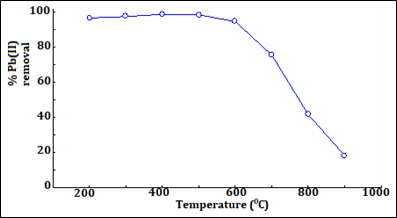Figure 2 Percent removal of Pb(II) ions from aqueous solution as a function of thermal treatment temperature of soil (sorbent dose =10 gL-1, pH = 5, Pb(II) ions concentration: 30 mgL-1, contact time = 6 hrs).

Specific surface area of sorbent

Specific surface areas of thermally treated soil sorbents (S3), determined using Sears’ method described in Section 2.3.5, was 32.4 m2/g.

XRD analysis

A typical XRD pattern of untreated soil (S3) is shown in Figure 3 and the percentage composition of various minerals present in the soil are recorded in Table 2. Whereas, Kaolinite [Al2Si2O5(OH)4] with highest concentration (52.6%) was found to be the major constituent of the selected soil, the level of Vermiculite [Mg3Si4O10(OH)2] with 3.3% concentration, was the lowest. The other two minerals: Quartz (SiO2) and Phosphosiderite [(FePO4(H2O)2] present in the soil were 24.5% and 19.6%, respectively.Figure 3 The X-ray diffraction (XRD) pattern of untreated soil sample (S3).

 Mineral Chemical Formula Percentage (%) Quartz SiO2 24.5 Vermiculite Mg3Si4O10(OH)2 3.3 Kaolinite Al2Si2O5(OH)4 52.6 Phosphosiderite FePO4(H2O)2 19.6

Table 2 Percentage composition of various minerals present in untreated soil sample (S3) as obtained from XRD analysis

Point of zero charge (pzc) of soil (sorbent)

The plot of electrical potential (millivolts) as a function of pH of the solution is shown Figure 4. The pzc of the soil (sorbent) obtainable from the pH value that corresponds to zero electrical potential on the plot was found to be 4.6. There always exists a relationship between pzc value of the sorbent and its sorption capacity. Whereas, the sorption of a cationic specie at a pH higher than the pzc of the sorbent will be favoured, an anion’s sorption, on the other hand, will be favored at pH lower than the pzc of sorbent.33Figure 4 Plot of electrical potential ( millivolts) as a function of pH for untreated and thermally treated soils collected from Gelemso (S3).

Effects of parameters on metal ions sorption

Various parameters that may affect the extent of metal ions sorption onto the sorbent include: sorbent amount, pH of solution, metal ions initial concentration, and sorbate-sorbent contact time.

Effect of sorbent amount

Percentage removal of Pb(II) ions from solution, and the amount of Pb(II) ions sorbed per unit mass of the sorbent qe (mgg-1) at sorbate-sorbent contact time 3 hrs, as a function of sorbent dose using initial concentration of Pb(II) = 20 mgL-1, solution pH = 5, are presented in Figure 5. Upon increasing sorbent concentration from 1 to 4 gm per 100 mL the percent removal of Pb(II) slightly increases, and at still higher sorbent load it becomes constant. The initial increase in the sorption of Pb(II) ions upon increasing the sorbent dose may be ascribed to enhanced availability of binding sites on the sorbent per sorbate ion/molecule.34 However, beyond the optimum value 4% (w/v) of sorbent dose, no additional removal of the sorbate was recorded indicating that the full sorption potential of the sorbent is being exploited.35 Further, with Pb(II) ions fixed initial concentration (20mgL-1), the sorption capacity i.e. the amount of Pb(II) ions sorbed per unit mass of the sorbent qe (mgg-1) at 3 hrs, decreased upon increasing sorbent dose.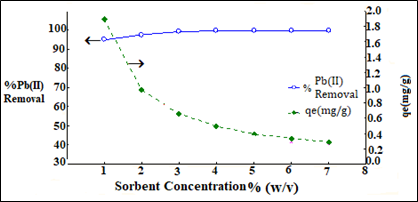Figure 5 Percentage removal of Pb(II) ions and amount of Pb(II) sorbed per unit mass of the sorbent at equilibrium, qe (mgg-1) as a function of sorbent dose (gL-1) (Pb(II) ions Initial concentration = 20 mgL-1, solution pH = 5, and sorbate-sorbent, contact time 3 hrs).

Effect of pH

The plot of % removal of Pb(II) ions at 2 hours, as a function of pH using Pb(II) ions initial concentration = 40 mg/L and sorbent dose 4 g per 100 mL is shown in Figure 5. Solution pH plays an important role in the sorption of the sorbate [(Pb(II) ions] due to its influence on the surface properties of the sorbent as well as on the ionic forms of the sorbate in solutions36 resulting variation in sorbate-sorbent interaction and hence the extent of the sorbate removal. For instance, at a pH < pzc (4.6) of sorbent, clay particles present in the soil interact with water in aqueous solution and acquire positive charge due to the formation of an aqua complex according to the following chemical reaction.37

Thus, the observed poor % removal of Pb(II) ions at low pH (Figure 6) can be due to the ion-ion repulsion due to positive charge both on the sorbent as well as the Pb(II) ions. However, at a pH >pzc, the sorbent’s surface becomes negatively charged as per the following chemical reactions38 favoring closer approach of the oppositely charged sorbent and the sorbate and thus the more % removal of the Pb(II) from the aqueous solution (Figure 6).Figure 6 Percent Pb(II) ions removal as a function of pH at 2 hours using Pb(II) ions initial concentration = 40 mg/L, adsorption dose = 4% (w/v).

Upon raising pH from 1 to 4, the removal of Pb(II) ions from aqueous solution increases due to diminishing positive charge on the sorbent surface at higher pH resulting in an enhanced sorbate -sorbent attraction. However, the observed constant percent removal of Pb(II) above pH 4, may be attributed to the saturation of by the binding sites on the sorbent as well as the attainment of sorption  desorption equilibrium.

Effect of contact time

In order to optimize the time required for maximum sorption of Pb(II) ions onto the sorbent, a plot of amount of Pb(II) ions sorbed per gram of sorbent, q (mgg-1), as a function of sorbate-sorbent contact time, using a fixed Pb(II) ions initial concentration 100 mgL-1; solution pH 4, and sorbent load 4% (w/v), was drawn (Figure 7). It was found that the metal ion sorption increased with increasing contact time till 120 min and beyond that it became constant. The observed increase in sorption of Pb(II) ions upto120 min (the optimum time), is because of higher rate of sorption compared to the desorption of the sorbate, and the subsequent constant metal ions sorption till 270 min is attributed to the attainment of the sorption desorption equilibrium. The amount of sorbate i.e. Pb(II)]ions, sorbed at the equilibrium time gives the maximum sorption capacity of the sorbent under the given condition.Figure 7 Plot of amount of Pb(II) ions sorbed per gram of sorbent, q (mgg-1) versus sorbate-sorbent contact time. [Pb(II) ions initial concentration =100 mgL-1; solution pH = 4, and sorbent load=4 % (w/v).

Effects of sorbate initial concentration

A plot of sorption capacity [amount of sorbate sorbed per gram of the sorbent, qe (mgg-1)] as a function of sorbate initial concentration (mgL-1) at specified conditions is shown in Figure 8. The sorption capacity was found increasing linearly, with the initial concentration of sorbate i.e, Pb(II) ions upto 100 mgL-1.Figure 8 Plot of sorption capacity [amount of (Pb(II) ions sorbed per gram of the sorbent, qe (mgg-1)] as a function of Pb(II) ions initial concentration (mgL-1) (solution pH = 4, sorbent load=4 % (w/v), and contact time 120 min).

Langmuir sorption isotherm

In Langmuir’s sorption treatment.39 It is assumed that a: sorbent surface is homogeneous; b: all active sites at the sorbent surface are equivalent; c: there is a monolayer coverage by the sorbate molecules at the sorbent surface, and d: there is no interaction between the sorbate molecules at adjacent binding sites. Accordingly, the linear form of the Langmuir sorption isotherm is given by the equation:

(5)

Where, ce is the equilibrium concentration of sorbate (mgL-1) in the solution,  is the amount of sorbate sorbed per unit mass of the sorbent at equilibrium (mgg-1); Qo is the amount of the sorbate sorbed per unit mass of sorbent (mgg-1) to form a monolayer, and KL is the Langmuir constant related to the affinity of binding sites (mg-1) and is a measure of the energy of sorption. The Langmuir constants Qo and KL obtained from the intercept and slope of linear plot of ce/qe versus ce (Figure 9) and are given in Table 3 along with the correlation coefficient (R2). The obtained high value of Langmuir parameter, KL =10.57 (mg)-1, suggests strong interaction of Pb(II) ions with the sorbent’s binding sites due to coulombic ion-ion interaction suggesting the involvement of a chemisorption process. Further, the value of parameter Qo = 2.215 (mgg-1) obtained, suggests a high intensity of Pb(II) ion sorption on the sorbent.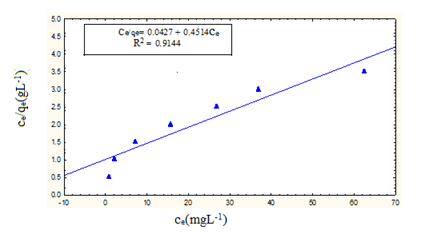Figure 9 Langmuir adsorption isotherm i.e. a plot of Ce/qe versus Ce [Pb(II) ions initial concentration = 20 mg L-1; pH = 4.5; sorbate-sorbent contact time = 120 min].

 Langmuir isotherm Freundlich isotherm Temkin isotherm Ce/qe = 1/(QoKL)+(1/Qo)Ce ln qe = ln KF + (1/n) lnCe qe = (RT/b)lnA + (RT/b)lnce Where, RT/b =B R2  = 0.9144 R2 = 0.9894 B= 0.3809 (L/g) Ce/qe = 1/(QoKL)+(1/Qo)Ce ln qe = ln KF + (1/n) lnCe qe = (RT/b)lnA + (RT/b)lnce Where, RT/b =B R2  = 0.9144 R2 = 0.9894 B= 0.3809 (L/g)

Table 3 Parameters of sorption isotherm models

Freundlich sorption isotherm

Freundlich sorption model40 assumes specific interactions of sorbate molecules/ions with the heterogeneous sites at the sorbent surface with varied affinities and also allows multilayer sorption. This model is more general than the Langmuir isotherm, since it does not assume a homogenous surface or constant sorption potential. It also assumes that the stronger binding sites are occupied first and their binding strength decreases with the increasing degree of site occupation. The Freundlich equation that describes sorbate-sorbent equilibrium is expressed as:

(6)

Where, qe represents the amount of solute retained by the sorbent (mg g-1), ce (mgL-1) is the solute equilibrium concentration in solution, Kf (Lmg-1) is related to the sorption capacity of the sorbent or bonding energy for a given sorbate - sorbent system at a given temperature and can be defined as the sorption or distribution coefficient representing the quantity of sobate sorbed for a unit equilibrium concentration, and ‘n’ is the dimensionless reaction order commonly less than one. It is also related to the sorption intensity or surface heterogeneity of the system. Freundlich model can be expressed by a linear form of equation.41

(7)

The values of Freundlich parameters KF and n, obtainable from the intercept and the slope, respectively, of the linear plot of logqe versus ln ce (Figure 10), are given in Table 3. The values of KF and ‘n’ thus obtained were 1.906 and 2.17, respectively. Since the value of “n” lies between 0 and 1, it indicates that the studied clay-rich soil can effectively adsorb the Pb(II) ions and thus can enable their removal from aqueous solution. The observed higher correlation coefficient (R2) for Freundlich model (0.9894) compared to that for Langmuir model (0.9144) suggests that the former model fits better for the studied sorbate-sorbent system.Figure 10 Freundlich isotherm i.e. a plot of log qe versus log ce for sorption of Pb(II) ions onto thermally treated clay-rich soil S3 [Pb(II) ions initial concentration = 20 mgL-1; pH = 4.5 ; sorbate-sorbent contact time = 120 min].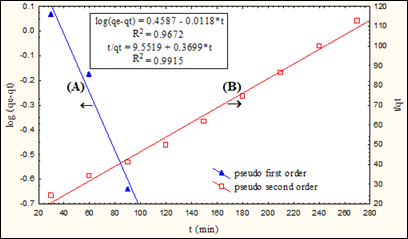Figure 10A Plot of log(qe - qt) versus contact time t (min), and (B): Plot of t/qt versus contact time t (min) [Pb(II) ions initial concentration 100mgL-1; sorbent load = 4 gL-1,pH = 5).

Temkin sorption isotherm

The sorption equilibrium data was also analyzed according to Temkin isotherm.42,43 The linear form of the Temkin isotherm can be expressed as:

(8)

Where, qe represents the amount of solute retained by the sorbent (mg g-1), ce (mgL-1) is the sorbate equilibrium concentration in solution, and RT/b=B. The parameters A and B determined from the intercept and slope of the plot qe versus lnce (Figure 11), are given, along with correlation co-efficient (R2), in Table 3. The correlation co-efficient for Temkin isotherm plot is lower than that for the Freundlich Isotherm but higher compared to the Langmuir Isotherm value Figure 11A.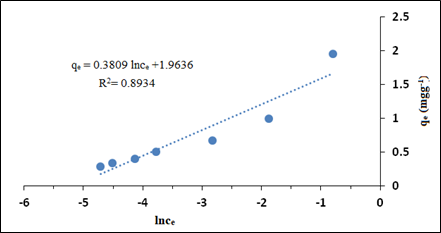Figure 11 Temkin sorption isotherm i.e. a plot of qe as a function lnc.Figure 11A The plot of amount of Pb(II) sorbed per gram of sorbent, qt(mgg-1) as a function of t1/2 ( Pb(II) ions initial concentration: 100 mgL-1, sorbent dose = 4 gL-1, pH = 5).

Sorption kinetic study

Three sequential steps involved during the sorption of a solute onto a sorbent are: 1. Film diffusion, where sorbate travels from the bulk towards the external surface of the sorbent; 2. Particle diffusion, where sorbate travels within the pores of the sorbent, and 3. Sorption of the sorbate on the interior surface of the sorbent.44 The observed sorption data were analyzed in terms of five different kinetic models as described in the following sections.

Pseudo-first-order kinetic model

The linear form of the pseudo-first-order sorption rate equation, can be written as:

(9)

or   (10)

Where, qe and qt are the theoretical sorption efficiency (amount of the sorbate i.e. Pb(II) ions, sorbed per gram of the sorbent (mgg-1) at equilibrium, and at time ‘t’, respectively; k1 is the sorption rate constant (min-1). The values of qe and k1, obtainable from the intercept and the slope, respectively, of the plot between log (qeqt) versus ‘t’ (Fig. 10A), are given along with the correlation coefficient (R2), in Table 4. The lower value of the calculated sorption efficiency qe (1.66 mgg-1) compared to the experimental qe (2.41mgg-1), suggests the poor applicability of the pseudo-first-order kinetic model to the studied sorbate-sorbent system.

 Experimental qe Sorption Kinetic Model Kinetic Parameters 2.41mgg-1 Pseudo-First-Order Kinetic Model log (qe – qt) = logqe – (k1/2.303)t qe = 1.29 mgg-1 k1 = 2.72x10-2 min-1 R2 = 0.9670 2.41mgg-1 Pseudo-Second-Order Kinetic Model t/qt = 1/(k2.qe2) + (1/qe).t k2 = 1.44x10-2 min-1 R2 = 0.9915 2.41mgg-1 Intra-particle diffusion Model qt = kit1/2+C ki = 9.73x10-2 min-1 C=1.0384 R2 = 0.7325 2.41mgg-1 Boyd Kinetic Model Bt = -0.4977 – ln(1-F) R2 = 0.8510 2.41mgg-1 The Elovich Model qt = (1/β)lnt + (1/β)ln (αβ) α = 3.72x10-2 min-1 β = 3.81 R2 = 0.9914

Table 4 Summary results of sorption kinetic models parameters

Pseudo-second-order kinetic model

The pseudo-second-order sorption rate can be represented by the linear form of equation:

(11)

Where, k2 is pseudo-second order sorption rate constant (g/mg min); qt and qe are amount of sorbate i.e Pb (II), sorbed per gram of the sorbent (mg/g) at contact time t, and at equilibrium, respectively. The values of qe, and k2, obtainable from the slope and intercept, respectively, of the linear plot of t/qt versus contact time t (Fig. 10B), are presented given in Table 4, along with the correlation coefficient (R2). The calculated qe value (2.70 mgg-1) close to the experimentally obtained qe (2.41mgg-1), and high correlation coefficient R2 =0.9910 imply that the sorption of Pb(II) onto the studied sorbent fits well in the pseudo-second-order sorption model.

Intra-particle diffusion model

Intra-particle diffusion model due to45 for a sorption system is generally expressed in the linear form of equation:

(12)

Where, qt is quantity of solute sorbed at the sorbent (mgg-1) at time t and ki­ is intra-particle diffusion rate constant (mg/g min1/2). The plot of qt as a function of t1/2 (using Pb(II) initial concentration 100 mgL-1, sorbent dose = 4 gL-1, pH = 5) is shown in in Figure 11. The values of ki and C were obtained from the slope and intercept, respectively, of qt versus t1/2 plot and are given in Table 4. Larger the value of C, greater is the boundary layer effect. For pure intra-particle diffusion to take place, the plot of qt versus t1/2 should be linear, passing through the origin. However, if the plot shows multilinearity, as is observed in the present case, it indicates that more than one step is involved in the sorption process i.e the sorption may be controlled by the combination of film and intraparticle diffusion46 Thus in the present case, it can be concluded that both the surface sorption as well as the intra-particle diffusion may be concurrently operating during the sorption of Pb(II) ions on the studied clay-rich soil sorbent (S-3). The intra-particle diffusion rate constant (ki = 9.73x10-2 min-1) was found to be higher than the sorption kinetic rate constants for pseudo -first- order (k1=2.72x10-2 min-1) as well as pseudo-second-order (k2=1.44x10-2 min-1).

The Boyd kinetic model

To determine the rate-controlling step involved in the sorption process, the observed data were also analyzed in terms of Boyd kinetic model47 represented by the following equation:

(13)

Where, F represents the fraction of solute sorbed at any time, t (min) and is given by F = qt/q. Where, qt, and q represent amount of the sorbate sorbed per gram of the sorbent (mg g-1) at time t (min) and at infinite time, respectively. The plot of Bt as a function of time t (min) is shown in Figure 12. The linearity test of Bt vs. time plot was used to distinguish between the film diffusion- and particle diffusion-controlled sorptions. The linear plot of Bt versus. time t passing through the origin, will suggest that the sorption rate is governed by particle diffusion mechanism otherwise the same is considered to be governed by film diffusion48 Since for the present sorbate-sorbent system the Bt versus time linear plot does not pass through the origin, it suggests that the sorption rate of Pb(II) ions onto the studied sorbent is controlled by film diffusion.Figure 12 Plot of Bt as a function of sorbate-sorbent contact time t(Pb(II) ions initial    concentration: 100 mgL-1, sorbent dose = 4 gL-1, pH = 5).

The Elovich kinetic model

The Elovich model of sorption kinetics49 can be represented by the equation: (βqt)

(14)

The integration of the Elovich rate equation using the same boundary conditions as for the pseudo-first-order, and pseudo second-order equations yields the linear form of Elovich Equation represented by:

qt = (1/β)lnt + (1/β)ln (αβ) (15)

Where, α is the initial sorption rate (mg/g min) and the parameter β (g/mg) is related to the extent of surface coverage and activation energy for chemisorptions.

The sorption kinetic constants α, and β, obtainable from the intercept and the slope of the linear plot of qt versus lnt (Figure 13) are given alongwith correlation coefficient (R2) in Table 4. The kinetic rate constant (α = 3.72x10-2 min-1) predicted by the Elovich equation is higher compared to sorption rates obtained for the pseudo -first- order (k1=2.72x10-2 min-1) and pseudo-second-order (k2=1.44x10-2 min-1) kinetic models. The correlation co-efficient R2 (= 0.9914) for Elovich equation is almost equal to that for the pseudo second order equation but higher than that for the other three studied kinedic models. Although Elovich Equation does not provide any mechanistic evidence, yet it has proved suitable for kinetic study of highly heterogeneous systems such as the present sorption of Pb (II) onto the clay-rich soil sorbent (S3).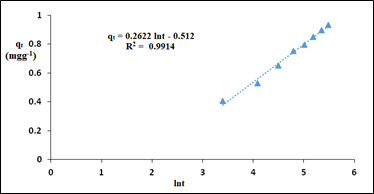Figure 13 Plot of qt(mgg-1)as a function of lnt [(Pb(II) ions initial concentration: 100 mgL-1, sorbe nt dose = 4 gL-1, pH = 5].

# Conclusion

The effects of sorbent dose, contact time, pH, and sorbate initial concentration on the sorption of Pb(II) ions on a clay-rich thermally treated soil, collected from a site near Gelemso town in Western Hararghie Zone of Oromia region of Ethiopia, have been studied using batch experiments. The sorption data were analyzed in terms of Langmuir, Freundlich and Temkin isotherms. The Freundlich sorption model was demonstrated to provide the best correlation for the studied sorbate-sorbent system indicating the existence of heterogeneous functional groups in the sorbent for interacting with Pb(II) ions. As high as 95% Pb(II) ions could be removed from their 20 mg/L-1 aqueous solution within 3 hrs using optimized pH and sorbent doze. Among the five kinetic models applied for the analysis of sorption data, the pseudo-second-order model, with highest R2 (= 0.9910), provided the best correlation with the experimental data. The results of present investigation show that studied clay-rich soil with sorption capacity 2.22 mgg-1 can be used as a low-cost sorbent for the treatment of wastewater contaminated with low levels of Pb(II) ions.

# Acknowledgments

The authors thankfully acknowledge the receipt of financial grants from the Ministry of Education, Ethiopia, through Haramaya University, for carrying out this research work and also appreciate the co-operation received from the Geosciences Laboratory of Geological Survey of Ethiopia, Addis Ababa, for their providing X-ray analysis facility.

# Conflicts of interest

The authors declare that there is no conflict of interest.

# References©2022 Fikre, et al. This is an open access article distributed under the terms of the, which permits unrestricted use, distribution, and build upon your work non-commercially.